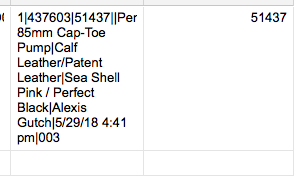# Beginner Formula Help - Extract Text from different cell

Hi,

Please excuse my beginner's questions. We are starting to use scanning QR codes into smart sheet. Currently our QR code translates to the below text, where important values are delimited by "|" symbol. When the below text is inserted in one cell, I'd like to create a formula that will extract the text after the second | and before the third |. So the extracted text from below would be 51437. Is there a combination of functions that can achieve this?

I hope this question makes sense.

1|437603|51437||Penelope 85mm Cap-Toe Pump|Calf Leather/Patent Leather|Sea Shell Pink / Perfect Black|Alexis Gutch|5/29/18 4:41 pm|003Tags:

• There is probably an easier way to do it, but this is what I have come up with thus far:

=RIGHT(LEFT([Column Name]@row, FIND("|", [Column Name]@row, VALUE(FIND("|", [Column Name]@row) + 1)) + 5), 5)

This will only pull the first 5 digits after the 2nd |. If it is possible that text is more or less than 5 digits, let me know and I'll see what I can figure out.

• This should get you everything between the 2nd and 3rd | regardless of length

[Column6]30 would be your cell with the QR Code

=LEFT(RIGHT([Column6]30, (LEN([Column6]30) - FIND("|", [Column6]30))), FIND("|", RIGHT([Column6]30, (LEN([Column6]30) - FIND("|", [Column6]30) - 1))))

• Sorry, that was beetween | 1 and |2

For |2 and |3 you would need

=LEFT(RIGHT(RIGHT([Column6]30, (LEN([Column6]30) - FIND("|", [Column6]30))),LEN(RIGHT([Column6]30, (LEN([Column6]30) - FIND("|", [Column6]30))))-FIND("|",RIGHT([Column6]30, (LEN([Column6]30) - FIND("|", [Column6]30))))),FIND("|", RIGHT(RIGHT([Column6]30, (LEN([Column6]30) - FIND("|", [Column6]30))),LEN(RIGHT([Column6]30, (LEN([Column6]30) - FIND("|", [Column6]30))))-FIND("|",RIGHT([Column6]30, (LEN([Column6]30) - FIND("|", [Column6]30))))))

## Help Article Resources

Want to practice working with formulas directly in Smartsheet?

Check out the Formula Handbook template!